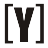# Scientific Calculator

Here is a scientific calculator complete with advanced features to calculate percentages, fractions, exponential functions, logarithms, trigonometry and more.

Scientific Calculator

0
mod
floor
ceil
(
)
C

%
+/-
sin
cos
tan
π
choose
7
8
9
÷
sin-1
cos-1
tan-1
e
2e
permute
4
5
6
×
x2
x3
xy
x-1
x!
1
2
3
-
3
x
log
ln
ex
rand
.
0
=
+

Others useful online calculators.YoosFuhl.com Would you like to receive notifications on latest updates? No Yes